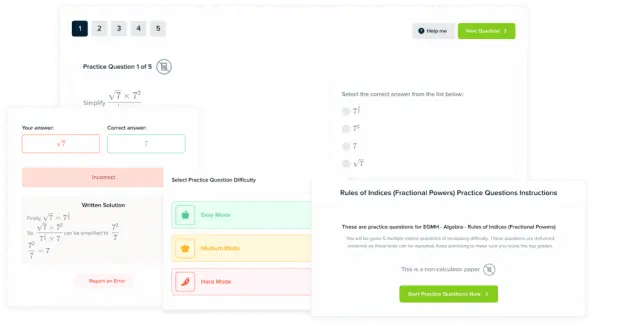# Rearranging Formulas

GCSEKS3Level 4-5Level 6-7AQACambridge iGCSEEdexcelEdexcel iGCSEOCRWJEC

## Rearranging Formulas

A formula is a way of giving a mathematical relationship between different things, expressed using algebra. For example, the relationship between speed, distance and time can be captured by the simple formula,

$s=\dfrac{{d}}{{t}}$.

You will need to be able to answer 5 key question types when rearranging formulas, these are shown below.

Make sure you are happy with the following topics before continuing.

Level 4-5GCSEKS3AQAEdexcelOCRWJECCambridge iGCSEEdexcel iGCSE

## Question type 1: Simple linear formula

The formula for the circumference of a circle is $C=2\pi r$. Here the circumference is the subject of the formula.

Making a different letter the subject of a formula means manipulating the formula to get that letter by itself on one side of the equation.

Example: For the equation $C=2\pi \textcolor{blue}{r}$, make $\textcolor{blue}{r}$ the subject.

Step 1: Dividing both sides of the equation by $2\pi$

\begin{aligned}(\textcolor{maroon}{\div 2\pi})\,\,\,\,\,\,\,\,\, C &= 2\pi \textcolor{blue}{r} \,\,\,\,\,\,\,\,\, (\textcolor{maroon}{\div 2\pi}) \\ \dfrac{C}{2\pi} &= \dfrac{\cancel{2\pi} \textcolor{blue}{r}}{\cancel{2\pi}} \end{aligned}

Step 2: Rewrite the formula in its final form, typically having the subject on the left hand side.

$\textcolor{blue}{r} = \dfrac{C}{2\pi}$

Level 4-5GCSEKS3AQAEdexcelOCRWJECCambridge iGCSEEdexcel iGCSE

## Question type 2: Formulas involving fractions

Some questions will include making a letter that appears in a fraction the subject of the formula.

$d=\dfrac{(u+v)t}{2}$

This formula describes the relation that distance, $d$, initial velocity, $u$, final velocity $v$ and time $t$ given constant acceleration. We can manipulate this formula to make $u$ the subject.

Example: For the equation $d=\dfrac{(\textcolor{blue}{u}+v)t}{2}$, make $\textcolor{blue}{u}$ the subject.

Step 1: Multiplying both sides by $2$,

\begin{aligned}(\textcolor{maroon}{\times 2})\,\,\,\,\,\,\,\,\, d & =\dfrac{(\textcolor{blue}{u}+v)t}{2} \,\,\,\,\,\,\,\,\, (\textcolor{maroon}{\times 2}) \\ 2d & =(\textcolor{blue}{u}+v)t \end{aligned}

Step 2: Dividing both sides of the equation by $t$,

\begin{aligned}(\textcolor{maroon}{\div t})\,\,\,\,\,\,\,\,\, 2d & =(\textcolor{blue}{u}+v)t \,\,\,\,\,\,\,\,\, (\textcolor{maroon}{\div t}) \\ \dfrac{2d}{t} & =\textcolor{blue}{u}+v\end{aligned}

Step 3: Finally subtracting $v$ and rewriting so $\textcolor{blue}u$ is on the left hand side,

\begin{aligned}(\textcolor{maroon}{-v})\,\,\,\,\,\,\,\,\, \dfrac{2d}{t} & =\textcolor{blue}{u}+v \,\,\,\,\,\,\,\,\, (\textcolor{maroon}{-v}) \\ \textcolor{blue}{u} & = \dfrac{2d}{t} -v\end{aligned}

Level 4-5GCSEKS3AQAEdexcelOCRWJECCambridge iGCSEEdexcel iGCSE
Level 4-5GCSEKS3AQAEdexcelOCRWJECCambridge iGCSEEdexcel iGCSE

## Question type 3: Formulas involving squares

Sometimes the required subject can appear as a square. For example, if we are asked to rearrange  the formula for the area of a circle to make $r$ the subject. Firstly, recall that the formula for the area of a circle is,

$A=\pi \textcolor{blue}{r^2}$

Example: For the equation $A=\pi \textcolor{blue}{r^2}$, make $\textcolor{blue}{r}$ the subject.

Step 1: Dividing both sides of the equation by $\pi$, we get,

$\dfrac{A}{\pi}=\textcolor{blue}{r^2}$

Now, $r$ is on its own on one side but it’s not technically the subject, since it is squared.

Step 2: If we now square root both sides of the equation, and since $r$ is always positive, we get,

$\textcolor{blue}{r}=\sqrt{\dfrac{A}{\pi}}$

Level 4-5GCSEKS3AQAEdexcelOCRWJECCambridge iGCSEEdexcel iGCSE

## Question type 4: Formulas involving square roots

Conversely, some questions may have the subject appear in a square root.

Example: Rearrange the following formula $d=\sqrt{\dfrac{3\textcolor{blue}{h}}{2}}$ to make $\textcolor{blue}{h}$ the subject.

Step 1: Squaring both sides of the equation, we get,

$d^2=\dfrac{3\textcolor{blue}{h}}{2}$

Step 2: Next, multiply both sides by $2$ to get,

$2d^2=3\textcolor{blue}{h}$

Step 3: Finally, dividing both sides by $3$ gives us,

$\textcolor{blue}{h}=\dfrac{2d^2}{3}$

Level 4-5GCSEKS3AQAEdexcelOCRWJECCambridge iGCSEEdexcel iGCSE
Level 6-7GCSEAQAEdexcelOCRWJECCambridge iGCSEEdexcel iGCSE

## Question type 5: Formulas when the subject appears twice

In some instances the required subject will appear more than once in the given formula. In these examples we factorise the terms involving the subject.

Example: Rearrange the following formula $H=2\textcolor{blue}{R}-g\textcolor{blue}{R}$ to make $\textcolor{blue}{R}$ the subject.

Step 1: We take out a factor of $R$ from both terms i.e. we factorise, and get

$H=\textcolor{blue}{R}(2-g)$

Step 2: Now we divide by $(2-g)$, to get

$\textcolor{blue}{R}=\dfrac{H}{2-g}$

Note: If your exams are with the OCR exam board, then you will need to know how to do this if you are a foundation student!

Level 6-7GCSEAQAEdexcelOCRWJECCambridge iGCSEEdexcel iGCSE

## Rearranging Formulas Example Questions

In order to make $m$ the subject, we will multiply both sides by $t$ and get:

$Ft=mv$

Then, if we divide both sides of the equation by $v$, and rewrite it so that $m$ is on the left hand side, we get,

$m=\dfrac{Ft}{v}$Gold Standard Education

Firstly, we will multiply both sides of the equation by 2 to get rid of the fraction:

$2A=(a+b)h$

Then, if we divide both sides by $h$, we get

$\dfrac{2A}{h}=a+b$

Finally, subtracting $b$ from both sides, we get

$a=\dfrac{2A}{h}-b$Gold Standard Education

Step 1: Multiplying both sides by $r^2$, we get

$Fr^2=kq$

Step 2: Next, divide both sides by $F$ to get

$r^2=\dfrac{kq}{F}$

Step 3: Finally, square rooting both sides gives us,

$r=\sqrt{\dfrac{kq}{F}}$Gold Standard Education

Multiplying both sides of the equation by $x+c$, we get

$x=\dfrac{a(x+c)}{b}$

Next, multiply both sides by $b$ and expanding the right-hand side, to get

$bx=ax+ac$

Subtracting $ax$ from both sides and factorising,

$bx-ax=x(b-a)=ac$

Finally, dividing both sides by $(b-a)$ gives us,

$x=\dfrac{ac}{b-a}$Gold Standard Education

Multiplying both sides of the equation by $b-4$, we get

$a(b-4)=(3-2b)$

Next, expanding the left-hand side, to get

$ab-4a=3-2b$

Collecting all the terms with a factor of $b$ on one side of the equation,

$ab+2b=3+4a$

Factorising $b$ out of the left-hand side and dividing by the terms left we find,

$b=\dfrac{3+4a}{a+2}$Gold Standard Education

## Rearranging Formulas Worksheet and Example Questions

### (NEW) Rearranging Formulas Exam Solutions - MME

Level 4-5GCSENewOfficial MME

## Rearranging Formulas Drill Questions

Level 4-5GCSE

### More Difficult Rearranging Formulae - Drill Questions

Level 6-7GCSEProduct

### £19.99

/month

Learn an entire GCSE course for maths, English and science on the most comprehensive online learning platform. With revision explainer videos & notes, practice questions, topic tests and full mock exams for each topic on every course, it’s easy to Learn and Revise with the MME Learning Portal.

## Related Topics

Level 1-3GCSEKS3

Level 4-5GCSEKS3

#### Expanding Brackets

Level 1-3Level 4-5GCSEKS3

#### Factorising

Level 4-5Level 6-7GCSE

#### Surds

Level 6-7Level 8-9GCSE